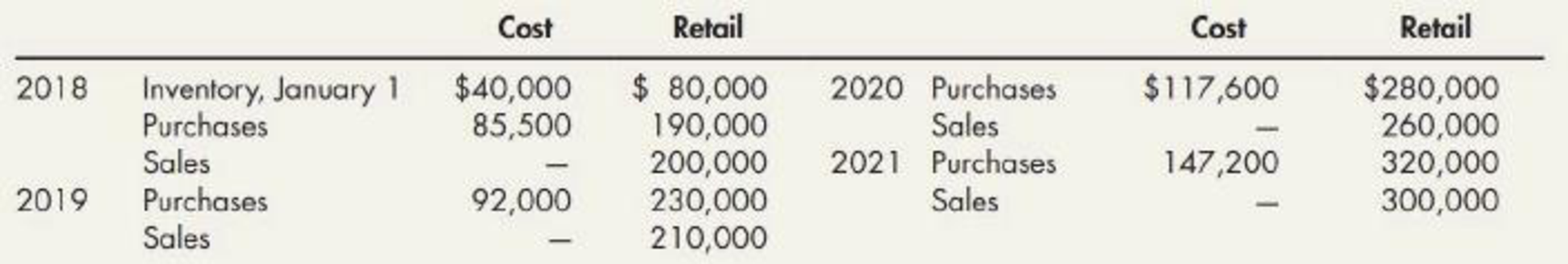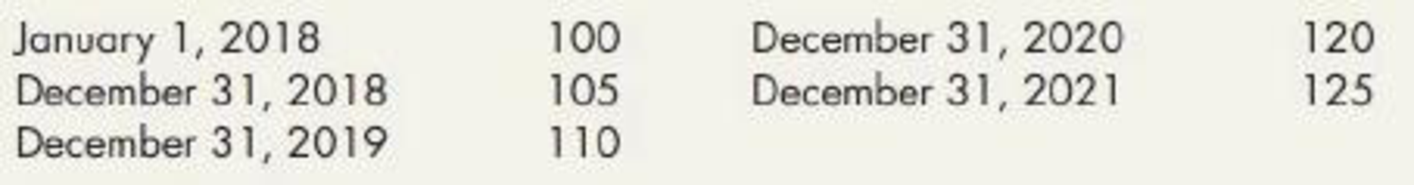Chapter 8, Problem 11P### Intermediate Accounting: Reporting...

3rd Edition
James M. Wahlen + 2 others
ISBN: 9781337788281

#### Solutions

Chapter
Section### Intermediate Accounting: Reporting...

3rd Edition
James M. Wahlen + 2 others
ISBN: 9781337788281
Textbook Problem
218 views

# Dollar-Value LIFO Retail Intella Inc. adopted the dollar-value retail LIFO method on January 1, 2018. The following data apply to the 4 subsequent years:In addition, the following price indexes are available:Required:Compute the inventory at the end of each of the 4 years. Round the cost-to-retail ratio to 3 decimal places.

To determine

Calculate the cost of ending inventory for 2018, 2019, 2020, and 2021 years by using dollar-value LIFO retail method.

Explanation

Dollar-Value-LIFO: This method shows all the inventory figures at dollar price rather than units. Under this inventory method, the units that are purchased last are sold first. Thus, it starts from the selling of the units recently purchased and ending with the beginning inventory.

Calculate the cost of ending inventory for 2018, 2019, 2020, and 2021 years by using dollar-value LIFO retail method:

For the year 2018:

Step 1: Calculate the amount of estimated ending inventory at retail.

 I Incorporation Ending Inventory Under DVL Retail Method For the Year 2018 Details Cost ($) Retail ($) Beginning inventory 40,000 80,000 Add:  Net purchase 85,500 190,000 Goods available for sale – Excluding beginning inventory 85,500 190,000 Goods available for sale – Including beginning inventory 125,500 270,000 Less:  Net sales (200,000) Estimated ending inventory at retail for 2018 $70,000 Table (1) Step 2: Calculate ending inventory at retail at base-year prices. Ending inventory at retail at base-year prices }=[ Ending inventory at retail×(Price index on 1/1/18Price index on 31/12/18)]=[$70,000×(100105)]=$66,667 Step 3: Calculate inventory change at retail at base year prices. Inventory change at retail at base-year prices}(Ending inventory at retail at base-year pricesBeginning inventory at retail)=($66,667$80,000)=$13,333

Step 4: Calculate the change at retail at relevant current costs.

Change at retail at relevant current costs =[Inventory change at retail at base-year prices×(Price index on 1/1/18Price index on 1/1/18)]=[$13,333×(100100)]=$13,333

Step 5: Calculate the change at relevant current costs.

Change at relevant current costs =[Change at retail at relevant current costs××Cost-to-retail ratio]=[$13,333×.50]=$6,667

Step 6: Calculate ending inventory at cost.

Ending inventory at cost = (Beginning inventory for 2018 Change at relevant current costs)=[$40,000$6,667]=$33,333 Hence, the ending inventory at cost for 2018 is$33,333.

Working note 1:

Calculate cost-to-retail ratio.

Cost-to-retail ratio= (Beginning inventory for costBeginning inventory for retail)=($40,000$80,000)=.5

Working note 2:

Calculate cost-to-retail ratio.

Cost-to-retail ratio= (Goods available for sale at retail excluding beginning inventoryGoods available for sale at retail excluding beginning inventory)=($85,500$190,000)=.45

For the year 2019:

Step 1: Calculate the amount of estimated ending inventory at retail.

 I Incorporation Ending Inventory Under DVL Retail Method For the Year 2019 Details Cost ($) Retail ($) Beginning inventory 33,333 70,000 Add:  Net purchase 92,000 230,000 Goods available for sale – Excluding beginning inventory 92,000 230,000 Goods available for sale – Including beginning inventory 125,333 300,000 Less:  Net sales (210,000) Estimated ending inventory at retail for 2019 $90,000 Table (1) Step 2: Calculate ending inventory at retail at base-year prices. Ending inventory at retail at base-year prices }=[ Ending inventory at retail×(Price index on 1/1/18Price index on 31/12/19)]=[$90,000×(100110)]=$81,818 Step 3: Calculate inventory change at retail at base year prices. Inventory change at retail at base-year prices}(Ending inventory at retail at base-year prices for 2019Ending inventory at retail at base-year prices for 2018)=($81,818$66,667)=$15,151

Step 4: Calculate the change at retail at relevant current costs.

Change at retail at relevant current costs =[Inventory change at retail at base-year prices×(Price index on 31/12/19Price index on 1/1/18)]=[$15,151×(110100)]=$16,666

Step 5: Calculate the change at relevant current costs.

Change at relevant current costs =[Change at retail at relevant current costs××Cost-to-retail ratio]=[16,666×.40]=$6,666 Step 6: Calculate ending inventory at cost. Ending inventory at cost = (Beginning inventory for 2019 +Change at relevant current costs)=[$33,333+$6,666]=$39,999

Hence, the ending inventory at cost for 2019 is $39,999. Working note 1: Calculate cost-to-retail ratio. Cost-to-retail ratio= (Goods available for sale at retail excluding beginning inventoryGoods available for sale at retail excluding beginning inventory)=($92,000\$230,000)=

### Still sussing out bartleby?

Check out a sample textbook solution.

See a sample solution

#### The Solution to Your Study Problems

Bartleby provides explanations to thousands of textbook problems written by our experts, many with advanced degrees!

Get Started

#### Find more solutions based on key concepts